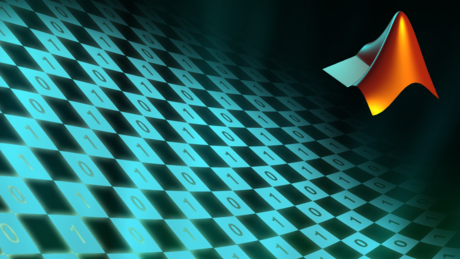# MATLAB Programming

Pioneered by University of New Mexico’s computer science chairman Cleve Moler in the early 1970s, MATLAB (short for matrix laboratory) is computer programming language that supports mathematical and numerical computations, as well as matrix manipulations, function plotting, data plotting, algorithm implementation, user interface creation and more. It can be used in conjunction with other popular languages like C, C++, Java and Python.

Moler had originally created MATLAB with the goal of providing his students access to LINPACK and EISPACK. It wasn’t long before the language was picked up by other schools — and other developers began tinkering with it to improve its utility. In the 1980s, Moler teamed up with engineer Jack Little and Steve Bangert to rewrite the language in C.

### MATLAB Features

MATLAB is loaded with features, many of which aim to streamline the otherwise complex and tedious processes of handling mathematical and numerical computations. It supports vector and matrix creations, engineering graphic creations, extensions in the form of Tool Boxes, File I/O functions, string processing, and object-oriented programming.

Furthermore, MATLAB is available on a wide variety of platforms, including DEC Alpha, HP 9000, IMB RS/6000, PC, MAC, Open VMS, Silicon Graphics and Sun Sparc. Nontetheless, MATLAB has become the de-facto standard language for mathematics courses in many universities and schools.

As explained on the University of Wisconsin-Madison website, the MATLAB system consists of five components: the MATLAB language, the MATLAB environment, the MATLAB graphics system, the MATLAB function library, and the MATLAB application program interface (API).

### What is MATLAB Used for?

One of the great things about MATLAB is its versatility. The fourth-generation proprietary programming language is used for a wide variety of applications, some of which include the following:

• Developing algorithms
• Math computations
• Presenting models
• Simulations
• Data analysis
• Scientific graphics
• Application development
• Graphical user interfaces
• And much more…

### MATLAB Alternatives

Of course, MATLAB isn’t the only computer programming language with a focus on mathematical and numerical computations. It has several paid/premium competitors, such as Mathmetica, Maple and TK Solver, as well as free and open-source alternatives like GNU Octave, Scilab, Julia and Sage.

### Did You Know?

There are numerous easter eggs hidden within the MATLAB language. If you type the word “spy,” for instance, MATLAB returns a picture of spies from the TV show Spy vs Spy. And typing in “why” results in a random philosophical answer. There are dozens of other easter eggs buried in MATLAB, offering a certain entertaining value that’s not found in other languages.